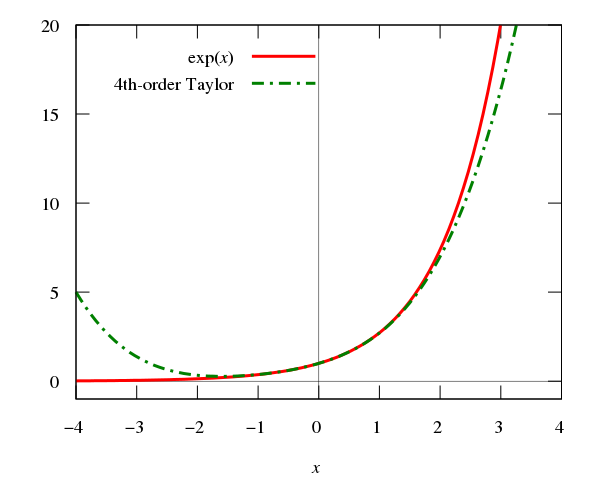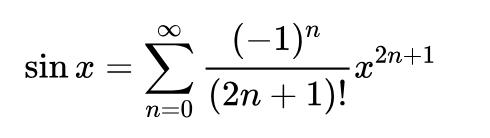# 简单介绍# 用程序计算 sin``````def factorial(n):
if n in (0, 1):
return 1
else:
return n * factorial(n - 1)

def sin(x):
i = 1
f = 1.0
sign = 1
sum = x
result = sum
while abs(sum) > 1e-10:
sign = -sign
f = f * (i + 1) * (i + 2)

i += 2
sum = sign * ((x ** i) / f)
result += sum
return result

if __name__ == '__main__':
import math
print sin(10)
print math.sin(10)
``````

# 参考资料Toc
Tags